# Determine the force in each member of the Pratt roof truss shown. State whether each member is in tension or compression.

Question-AnswerCategory: Engineering MechanicsDetermine the force in each member of the Pratt roof truss shown. State whether each member is in tension or compression.

Determine whether the block shown is in equilibrium and find the magnitude and direction of the friction force when θ = 40° and P = 400 N.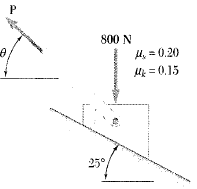Step: 1

Draw the free body diagram of the body as follows: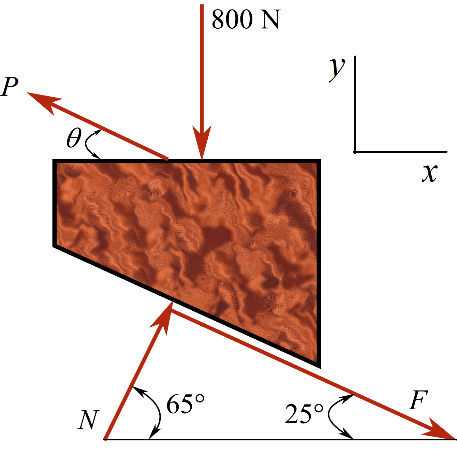Step: 2

Apply the force equilibrium condition about x-axis.Substitute 400 N for P andfor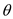.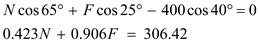…… (1)
Apply the force equilibrium condition about y-axis.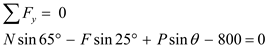Substitute 400 N for P and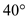for.…… (2)

Step: 3

Substitute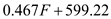for N in the equation (1).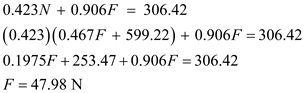Substitute 47.98 N for F in the equation (2).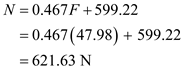Step: 4

Calculate the maximum frictional force.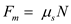Here,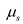is the coefficient of static friction.
Substitute 0.20 for.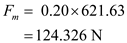Since,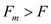, the block is in equilibrium.
Therefore, the frictional force is.